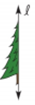Chapter 2.6, Problem 11E### Elementary Geometry for College St...

6th Edition
Daniel C. Alexander + 1 other
ISBN: 9781285195698

#### Solutions

Chapter
Section### Elementary Geometry for College St...

6th Edition
Daniel C. Alexander + 1 other
ISBN: 9781285195698
Textbook Problem
1 views

# Complete each figure so that it has symmetry with respect toline l .To determine

a)

To draw:

The complete figure symmetrical to line 𝓁.

Explanation

Definition Used:

The figure to be symmetrical to the given line for every point A on the left side of the line, there should be second point A such that line should act as the perpendicular bisector of AA¯.

Approach:

The complete figure symmetrical to line 𝓁 is shown in given figure

To determine

b)

To draw:

The complete figure symmetrical to line 𝓁.

### Still sussing out bartleby?

Check out a sample textbook solution.

See a sample solution

#### The Solution to Your Study Problems

Bartleby provides explanations to thousands of textbook problems written by our experts, many with advanced degrees!

Get Started

#### Convert 3,400 feet to miles.

Trigonometry (MindTap Course List)

#### For , f(x) = 0 2 3 f(3) does not exist

Study Guide for Stewart's Multivariable Calculus, 8th

#### limx5x225x29x+20= a) 1 b) 10 c) d) does not exist

Study Guide for Stewart's Single Variable Calculus: Early Transcendentals, 8th